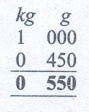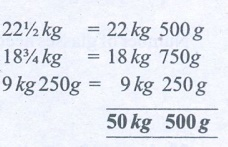Home | | Maths 6th Std | Exercise 2.1

# Exercise 2.1

6th Maths : Term 2 Unit 2 : Measurements : Exercise 2.1 : Text Book Back Exercises Questions with Answers, Solution

Exercise 2.1

1. Fill in the blanks

(i) 250 ml + ½ l¾ l

250 ml = ¼  l + ½ l   = ¾ l

(ii) 150 kg 200 g + 55 kg 750 g205 kg 950 g

(iii) 20 l – 1 l 500 ml18 l 500 ml(iv) 450 ml × 5 = 2 l 250 ml

450 ml × 5

2250 ml ÷ 1000 = 2l 250 l

(v) 50 kg ÷ 100 g500g50 kg (50000/100) = 500 g

2. True or False

(i) Pugazhenthi ate 100 g of nuts which is equal to 0.1 kg. [True]

(ii) Meena bought 250 ml of buttermilk which is equal to 2.5 l. [False]

(250 ml ÷ 1000 = 0.250l)

(iii) Karkuzhali’s bag 1 kg 250 g and Poongkodi’s bag 2 kg 750 g. The total weight of their bags 4 kg. [True]

(iv) Vanmathi bought 4 books each weighing 500 g. Total weight of 4 books is 2 kg. [True]

(v) Gayathri bought 1 kg of birthday cake. She shared 450 g with her friends. The weight of cake remaining is 650 g. [False]

[1 kg 000g ] – [0kg 450 g] = 0kg 550g3. Convert into indicated units:

(i) 10 l and 50 ml into ml

(ii) 4 km and 300 m into m

(iii) 300 mg into g

(i) 10 l and 50 ml into ml

10l 50 ml = (10 × 1000) ml + 50 ml. (1 l = 1000 ml)

= 10000 ml +50 ml

= 10050 ml

(ii) 4 km and 300 m into m

4 km 300 m = (4 × 300) m + 300 m (1 km = 1000 m)

= 4000 m + 300 m

= 4300 m

(iii) 300 mg into g

300 mg = 300 ÷ 1000 g (1000 mg = 1 g)

= 0.3 g

4. Convert into higher units :

(i) 13000 mm (km, m, cm)

(ii) 8257 ml (kl, l)

(i) 13000 mm (km, m, cm)

13000 mm = (13000 ÷ 10) cm = 1300 cm (10mm = 1 cm)

13000 mm = (13000 ÷ 1000) m = 13 m (1000 mm = l m)

13000 mm = (13000 ÷ 1000000) km

= 0.013 km (1000000 mm = 1 km)

(ii) 8257 ml (kl, l)

8257 ml = (8257 ÷ 1000) l = 8.257 l (1000 ml = 1 l )

8257 ml = (8257 ÷ 1000000) l = 0.008257 kl (1000000 ml = 1 kl)

5. Convert into lower units :

(i) 15 km (m, cm, mm)

(ii) 12 kg (g, mg)

(i) 15 km (m, cm, mm)

15 km = (15 × 1000000) mm = 15000000 mm

(1000000 mm = 1 km)

15 km = (15 × 100000) cm = 1500000 cm (100000 cm = 1 km)

15 km = (15 × 1000) m = 15000 m (1000 m = 1 km)

(ii) 12 kg (g, mg)

12 kg = (12 × 1000000) mg = 12000000 mg

(1000000 mg = 1 kg)

12 kg = (12 × 1000) g = 12000 g (1000 g = 1 kg)

6. Compare and put >or< or = in the following:

(i) 800 g + 150 g 1 kg

(ii) 600 ml+400 ml 1 l

(iii) 6 m 25 cm 600 cm+25 cm

(iv) 88 cm 8 m 8 cm

(v) 55 g 550 mg

(i) 800 g + 150 g [<] 1 kg

(ii) 600 ml + 400 ml [=] 1 l

(iii) 6 m 25 cm [=] 600 cm + 25 cm

(iv) 88 cm [<] 8 m 8 cm

(v) 55 g [>] 550 mg

7. Geetha brought 2 l and 250 ml of water in a bottle. Her friend drank 300 ml from it. How much of water is remaining in the bottle?

Water in a bottle = 2l 250 ml

Her friend drank water  = 0l  300 ml

Remaining water in the bottle = 1 l  950 ml

8. Thenmozhi’s height is 1.25 m now. She grows 5 cm every year.  what would be her height after 6 years?

Thenmozhi’s height now = 1.25 m = 125 cm

Height she grows in one year = 5 cm

Height she grows in 6 years = 6 × 5 = 30 cm

After 6 years her height = Now her height + 30 cm

= (125 + 30) cm

= 155 cm

After 6 years her height = 155 cm.

9. Priya bought 22 ½ kg of onion, Krishna bought 18 3/4 kg of onion and Sethu bought 9 Kg 250 g of onion.what is the total weight of onion did they buy?

Weight ofonion Priya bought = 22 ½   kg = 22 kg 500 g

Weight ofonion Krishna bought  = 18 ¾  kg = 18 kg 750g

Weight of onion Sethu bought = 9 kg 250g = 9 kg 250 gTotal weight of onion they bought = 50 kg 500g

10. Maran walks 1.5 km every day to reach the school while Mahizhan walks 1400 m. Who walks more distance and by how much?

Distance walks by Maran = 1.5 kg = 1500 m

Distance walks by Mahizhan = 1400 m = 1400 mMaran walks more distance = 100 m

11. In a JRC one day camp, 150 gm of rice and 15 ml oil are needed for a student. If there are 40 students to attend the camp how much of rice and oil are needed?

Rice needed for a student = 150g

Rice needed for 40 students = 150 × 40g = 6000 g = 6 kg

Oil needed for a students = 15 ml

Oil needed for 40 students = 15 × 40 ml = 600 ml = 0.6 l

Rice needed for 40 students = 6 kg

Oil needed for 40 students = 0.6 l

12. In a school, 200 litres of lemon juice is prepared. If 250 ml lemon juice is given to each student, how many students get the juice?

Amount of lemon juice prepared = 2001

= 200000 mlAmount of juice given to one student = 250 ml

Number of students get the juice = 200000 ÷ 250

= 800

Number of students get the juice = 800

13. How many glasses of the given capacity will fill a 2 litre jug?

(i) 100 ml_____

Capacity of jug = 21 = 2000 ml.

Capacity of a glass = 100 ml.

Number of glasses needed = Capacity of a jug / Capacity of a glass

= 2000 / 100

= 20

20 glasses of capacity 100 ml, will fill a 2 litre jug.

(ii) 50 ml_____

Capacity of a glass = 50 ml.

Number of glasses needed= Capacity of a jug / Capacity of a glass

= 2000 / 50 = 40

40 glasses of capacity 50 ml, will fill a 2 litre jug.

(iii) 500 ml____

Capacity of a glass = 500 ml.

Number of glasses needed = Capacity of a jug / Capacity of a glass

= 2000 / 500  = 4

4 glasses of capacity 500 ml, will fill a 2 litre jug.

(iv) 1 l_____

Capacity of a jug = 1 l.

Number of glasses needed = Capacity of a glass / Capacity of a glass

= 2 / 1 = 2

2 glasses of capacity 1 l, will fill a 2 litre jug.

(v) 250 ml _____

Capacity of a jug = 250 ml.

Number of glasses needed = Capacity of a glass / Capacity of a glass

= 2000 / 250 = 8

8 glasses of capacity 500 ml, will fill a 2 litre jug.

Objective Type Questions

14. 9 m 4 cm is equal to _________

a. 94 cm

b. 904 cm

c. 9.4 cm

d. 0.94 cm

15. 1006 g is equal to _________

a. 1 kg 6 g

b. 10 kg 6 g

c. 100 kg 6 g

d. 1 kg 600 g

Answer: a) 1 kg 6 g

16. Every day 150 l of water is sprayed in the garden.  Water sprayed in a week is _____

a. 700 l

b. 1000 l

c. 950 l

d. 1050 l

17. Which is the greatest 0.007 g, 70 mg, 0.07 cg

a. 0.07 cg

b. 0.007 g

c. 70 mg

d. all are equal

18. 7 km – 4200 m is equal to _________

a. 3 km 800 m

b. 2 km 800 m

c. 3 km 200 m

d. 2 km 200 m

Answer: b) 2 km 800 m

Exercise: 2.1

1. i. 3/4 l ii. 205 kg 950 g  iii. 18 l 500 ml  iv. 2 l 250 ml   v. 500

2. i. True   ii. False        iii. True  iv. True     v. False

3. i. 10005 ml         ii. 4300 m       iii. 0.3 g

4. (i) 1300 cm, 13 m, 0.013 km (ii) 8.257 l, 0.008257 kl

5. i) 15000 m, 1500000 cm, 15000000 mm

ii) 12000 g, 12000000 mg

6. i) < ii) = iii) = iv) < v) >

7. 1 l 950 ml

8. 155 cm

9. 50 kg 500 g

10. Maran ,100 m

11. 6 kg, 0.6 l

12. 800 students

13. i. 20 glasses ii. 40 glasses  iii. 4 glasses iv. 2 glasses  v. 8 glasses

Objective Type Questions

14. ( b) 904 cg

15. (a)1 kg 6 g

16. (d) 1050 l

17. (d) 70 mg

18. (b) 2 km 800 m

Tags : Questions with Answers, Solution | Measurements | Term 2 Chapter 2 | 6th Maths , 6th Maths : Term 2 Unit 2 : Measurements
Study Material, Lecturing Notes, Assignment, Reference, Wiki description explanation, brief detail
6th Maths : Term 2 Unit 2 : Measurements : Exercise 2.1 | Questions with Answers, Solution | Measurements | Term 2 Chapter 2 | 6th Maths